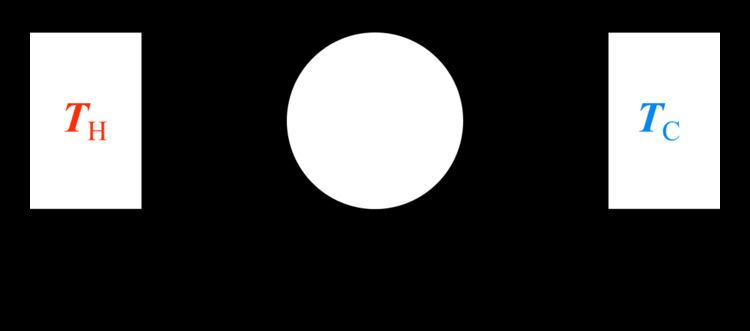# Thermodynamic state

Updated on
Covid-19For thermodynamics, a thermodynamic state of a system is its condition at a specific time, that is fully identified by values of a suitable set of parameters known as state variables, state parameters or thermodynamic variables. Once such a set of values of thermodynamic variables has been specified for a system, the values of all thermodynamic properties of the system are uniquely determined. Usually, by default, a thermodynamic state is taken to be one of thermodynamic equilibrium. This means that the state is not merely the condition of the system at a specific time, but that the condition is the same, unchanging, over an indefinitely long duration of time.

## Contents

Thermodynamics sets up an idealized formalism that can be summarized by a system of postulates of thermodynamics. Thermodynamic states are amongst the fundamental or primitive objects or notions of the formalism, in which their existence is formally postulated, rather than being derived or constructed from other concepts.

A thermodynamic system is not simply a physical system. Rather, in general, indefinitely many different alternative physical systems comprise a given thermodynamic system, because in general a physical system has vastly many more microscopic characteristics than are mentioned in a thermodynamic description. A thermodynamic system is a macroscopic object, the microscopic details of which are not explicitly considered in its thermodynamic description. The number of state variables required to specify the thermodynamic state depends on the system, and is not always known in advance of experiment; it is usually found from experimental evidence. Always the number is two or more; usually it is not more than some dozen. Though the number of state variables is fixed by experiment, there remains choice of which of them to use for a particular convenient description; a given thermodynamic system may be alternatively identified by several different choices of the set of state variables. The choice is usually made on the basis of the walls and surroundings that are relevant for the thermodynamic processes that are to be considered for the system. For example, if it is intended to consider heat transfer for the system, then a wall of the system should be permeable to heat, and that wall should connect the system to a body, in the surroundings, that has a definite time-invariant temperature.

For equilibrium thermodynamics, in a thermodynamic state of a system, its contents are in internal thermodynamic equilibrium, with zero flows of all quantities, both internal and between system and surroundings. For Planck, the primary characteristic of a thermodynamic state of a system that consists of a single phase, in the absence of an externally imposed force field, is spatial homogeneity. For non-equilibrium thermodynamics, a suitable set of identifying state variables includes some macroscopic variables, for example a non-zero spatial gradient of temperature, that indicate departure from thermodynamic equilibrium. Such non-equilibrium identifying state variables indicate that some non-zero flow may be occurring within the system or between system and surroundings.

## State functions

Besides the thermodynamic variables that originally identify a thermodynamic state of a system, the system is characterized by further quantities called state functions, which are also called state variables, thermodynamic variables, state quantities, or functions of state. They are uniquely determined by the thermodynamic state as it has been identified by the original state variables. A passage from a given initial thermodynamic state to a given final thermodynamic state of a thermodynamic system is known as a thermodynamic process; usually this is transfer of matter or energy between system and surroundings. In any thermodynamic process, whatever may be the intermediate conditions during the passage, the total respective change in the value of each thermodynamic state variable depends only on the initial and final states. For an idealized continuous or quasi-static process, this means that infinitesimal incremental changes in such variables are exact differentials. Together, the incremental changes throughout the process, and the initial and final states, fully determine the idealized process.

In the most commonly cited simple example, an ideal gas, the thermodynamic variables would be any three variables out of the following four: mole number, pressure, temperature, and volume. Thus the thermodynamic state would range over a three-dimensional state space. The remaining variable, as well as other quantities such as the internal energy and the entropy, would be expressed as state functions of these three variables. The state functions satisfy certain universal constraints, expressed in the laws of thermodynamics, and they depend on the peculiarities of the materials that compose the concrete system.

Various thermodynamic diagrams have been developed to model the transitions between thermodynamic states.

## Equilibrium state

Physical systems found in nature are practically always dynamic and complex, but in many cases, macroscopic physical systems are amenable to description based on proximity to ideal conditions. One such ideal condition is that of a stable equilibrium state. Such a state is a primitive object of classical or equilibrium thermodynamics, in which it is called a thermodynamic state. Based on many observations, thermodynamics postulates that all systems that are isolated from the external environment will evolve so as to approach unique stable equilibrium states. There are a number of different types of equilibrium, corresponding to different physical variables, and a system reaches thermodynamic equilibrium when the conditions of all the relevant types of equilibrium are simultaneously satisfied. A few different types of equilibrium are listed below.

• Thermal Equilibrium: When the temperature throughout a system is uniform, the system is in thermal equilibrium.
• Mechanical Equilibrium: If at every point within a given system there is no change in pressure with time, and there is no movement of material, the system is in mechanical equilibrium.
• Phase Equilibrium: This occurs when the mass for each individual phase reaches a value that does not change with time.
• Chemical Equilibrium: In chemical equilibrium, the chemical composition of a system has settled and does not change with time.
• Thermodynamic state Wikipedia

Topics